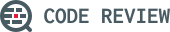## FizzBuzz on FizzBuzz

1

(click on this box to dismiss)Q&A for peer programmer code reviews

``````DECLARE @Question INT = (SELECT Id FROM PostTypes WHERE Name = 'Question');
DECLARE @FizzBuzzTag INT = 527;
DECLARE @FIZZ INT = 3;
DECLARE @BUZZ INT = 5;

SELECT DISTINCT
, [Postz Idz] = CASE
WHEN (Posts.Id % (@FIZZ*@BUZZ)) = 0 THEN 'FizzBuzz'
WHEN (Posts.Id % @FIZZ) = 0 THEN 'Fizz'
WHEN (Posts.Id % @BUZZ) = 0 THEN 'Buzz'
ELSE CAST(Posts.Id AS VARCHAR(MAX)) END
, [Scorez] = CASE
WHEN (Posts.Score % (@FIZZ*@BUZZ)) = 0 THEN 'FizzBuzz'
WHEN (Posts.Score % @FIZZ) = 0 THEN 'Fizz'
WHEN (Posts.Score % @BUZZ) = 0 THEN 'Buzz'
ELSE CAST(Posts.Score AS VARCHAR(MAX)) END
, [Userz Idz] = CASE
WHEN (Users.Id % (@FIZZ*@BUZZ)) = 0 THEN 'FizzBuzz'
WHEN (Users.Id % @FIZZ) = 0 THEN 'Fizz'
WHEN (Users.Id % @BUZZ) = 0 THEN 'Buzz'
ELSE CAST(Users.Id AS VARCHAR(MAX)) END
, [Userz Repz] = CASE
WHEN (Users.Reputation % (@FIZZ*@BUZZ)) = 0 THEN 'FizzBuzz'
WHEN (Users.Reputation % @FIZZ) = 0 THEN 'Fizz'
WHEN (Users.Reputation % @BUZZ) = 0 THEN 'Buzz'
ELSE CAST(Users.Reputation AS VARCHAR(MAX)) END
, [Creation Date] = Posts.CreationDate
, [Tags] = Posts.Tags
FROM PostsHold tight while we fetch your results